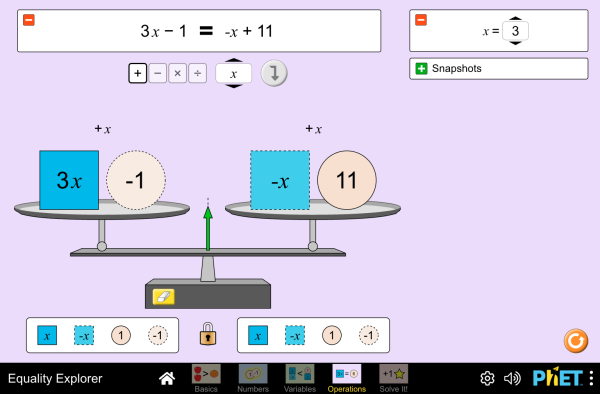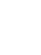# Istraživač jednačinaPreuzimanje (Download)Ugradnja (Embed) zatvori Ugradi (Embed) izvršnu kopiju ove simulacije Koristi ovaj HTML za ugradnju (embed) izvršne kopije ove simulacije. Možeš mijenjati dimenzije prikaza (širinu i visinu ekrana) mijenjajući "width" i "height" atribute u HTML. Ugradi sliku. Klikom na nju pokretat će se simulacija.
Klikni i započni
Koristi ovaj HTML kod da bi se pojavio ekran sa natpisom "Klikni i započni" ("Click to Run"). Solving Equations Inequalities Inverse Operations PhET je podržаn od:i nаstаvnikа kаo što ste Vi!

• Solving Equations
• Inequalities
• Inverse Operations

### Description

Explore what it means for a mathematical statement to be balanced or unbalanced by interacting with objects on a balance. Discover the rules for keeping it balanced. Collect stars by playing the game!

### Primjeri obrazovnih ciljeva

• Solve and manipulate algebraic equations by applying properties of real numbers (in particular, the inverse properties of addition and multiplication) and properties of equality
• Solve and manipulate algebraic equations by substituting different values for a variable
• Use a balance model to solve an equation for an unknown, and justify your strategies for solving
• Solve an equation using only universal operations

### Standards Alignment

#### Common Core - Math

6.EE.B.5
Understand solving an equation or inequality as a process of answering a question: which values from a specified set, if any, make the equation or inequality true? Use substitution to determine whether a given number in a specified set makes an equation or inequality true.
6.EE.B.6
Use variables to represent numbers and write expressions when solving a real-world or mathematical problem; understand that a variable can represent an unknown number, or, depending on the purpose at hand, any number in a specified set.
7.EE.B.3
Solve multi-step real-life and mathematical problems posed with positive and negative rational numbers in any form (whole numbers, fractions, and decimals), using tools strategically. Apply properties of operations to calculate with numbers in any form; convert between forms as appropriate; and assess the reasonableness of answers using mental computation and estimation strategies. For example: If a woman making \$25 an hour gets a 10% raise, she will make an additional 1/10 of her salary an hour, or \$2.50, for a new salary of \$27.50. If you want to place a towel bar 9 3/4 inches long in the center of a door that is 27 1/2 inches wide, you will need to place the bar about 9 inches from each edge; this estimate can be used as a check on the exact computation.
7.EE.B.4
Use variables to represent quantities in a real-world or mathematical problem, and construct simple equations and inequalities to solve problems by reasoning about the quantities.
8.EE.C.7
Solve linear equations in one variable.
8.EE.C.7a
Give examples of linear equations in one variable with one solution, infinitely many solutions, or no solutions. Show which of these possibilities is the case by successively transforming the given equation into simpler forms, until an equivalent equation of the form x = a, a = a, or a = b results (where a and b are different numbers).
8.EE.C.8
Analyze and solve pairs of simultaneous linear equations.
HSA-CED.A.1
Create equations and inequalities in one variable and use them to solve problems. Include equations arising from linear and quadratic functions, and simple rational and exponential functions.
HSA-CED.A.3
Represent constraints by equations or inequalities, and by systems of equations and/or inequalities, and interpret solutions as viable or nonviable options in a modeling context. For example, represent inequalities describing nutritional and cost constraints on combinations of different foods.
HSA-REI.A.1
Explain each step in solving a simple equation as following from the equality of numbers asserted at the previous step, starting from the assumption that the original equation has a solution. Construct a viable argument to justify a solution method.
HSA-REI.B.3
Solve linear equations and inequalities in one variable, including equations with coefficients represented by letters.
Version 1.0.12

### Teacher TipsVodič za nastavnike (pdf) sadrži savjete kreirane оd strane PhET tima. ( PDF ).

### Teacher-Submitted Activities

Games Remote Lesson ideasTrish Loeblein MS (SŠ)
K-5 (OŠ)
HS (VŠ)
UG-Intro (VSŠ)
Remote
DZ
Fizika
Matematika
Hemija
Introducción a la igualdadDiana López MS (SŠ)
HS (VŠ)
DZ
Lab
Guided
Matematika
Balancing ActMichael Reynolds MS (SŠ) Guided Matematika
What is EqualityDavid Wood MS (SŠ)
HS (VŠ)
Guided
Lab
Discuss
DZ
Matematika
Introduction to InequalitiesMarleene Buttice MS (SŠ) Guided Matematika
Solving Multi-Step EquationsMeenakshi Raghavan MS (SŠ) Guided Matematika
Middle School Math Sim AlignmentAmanda McGarry MS (SŠ) Ostalo Matematika
SECUNDARIA: Alineación PhET con programas de la SEP México (2011 y 2017)Diana López MS (SŠ)
HS (VŠ)
Ostalo Hemija
Biologija
Matematika
Fizika
PREPARATORIA: Alineación de PhET con programas de la DGB México (2017)Diana López HS (VŠ)
UG-Intro (VSŠ)
Ostalo Matematika
Fizika
Hemija
PRIMARIA: Alineación con programas de la SEP México (2011 y 2017)Diana López K-5 (OŠ)
MS (SŠ)
Demo
Discuss
DZ
Lab
Guided
Fizika
Hemija
Matematika
Astronomija
Equality Explorer - 6th Grade intro to solving Trevor Gibson MS (SŠ) Guided Matematika
Exploring Equations Akili Obika HS (VŠ)
MS (SŠ)
Guided Matematika
Equação e balança Alison Sousa da Silva, Ana Carolina Costa Pereira MS (SŠ) Lab
Guided
Matematika
Jezik Downloador RunTips
Albanski All shqipHulumtues barazishë
Arapski All العربيةمستكشف المساواة
Baskijski All EuskaraBerdintasun-esploratzailea
Brazilski Portugalski All português (Brasil)Explorador da Igualdade
Češki All češtinaPrůzkumník rovnosti
Danski All DanskLigevægts-visning
Engleski All EnglishEquality Explorer
Francuski All françaisExplorateur d'égalité
Grčki All ΕλληνικάΕξερευνητής εξισώσεων
Gujarati All Gujaratiસમીકરણ સમાનતા શોધક- Pankajsid34
Hindu All हिंदीसमानता का पता करें
Holandski All NederlandsOntdek gelijkheid
Hrvatski All hrvatskiIstraživanje jednadžbi
Italijanski All italianoEsplora le equazioni
Japanski All 日本語つり合いを作ろう
Kazaški All KazakhТеңсіздіктер шешімі
Kineski - Tradicionalni All 中文 (台灣)Equality Explorer_探索等式
Kineski - Uprošćen All 中文 (中国)等式探索
Korejski All 한국어등식 연습하기
Letonski All LatviešuVienādību pētīšana
Mađarski All magyarEgyenlőségvizsgálat
Mongolski All Монгол (Монгол)Тэнцэтгэл Судлах
Njemački All DeutschGleichheit schaffen
Persijski All فارسیکاوشگر برابری
Poljski All polskiPoznajemy równania
Portugalski All portuguêsExplorador de Igualdades
Ruski All русскийРешение неравенств
Slovački All SlovenčinaSkúmanie rovnosti
Španski All españolExplorador de IgualdadesŠpanski (Meksiko) All español (México)Explorador de IgualdadesŠpanski (Peru) All español (Perú)Explorador de Igualdad
Srpski All СрпскиИстраживач једначина
Švedski All svenskaUtforska likheter!
Turski All TürkçeEşitlik Gezgini
Ukrajinski All українськаДослідник рівностейVijetnamski All Tiếng ViệtPhương trình

HTML5 sims can run on iPads and Chromebooks, as well as PC, Mac, and Linux systems.

iOS 12+ Safari

Android:
Not officially supported. If you are using the HTML5 sims on Android, we recommend using the latest version of Google Chrome.

Chromebook:
The HTML5 and Flash PhET sims are supported on all Chromebooks.
Chromebook compatible sims

Windows Systems:

Macintosh Systems:

Linux Systems:

Dizajn tim Nezavisne baze i biblioteke Hvala:
• Chris Malley (developer)
• Diana López Tavares
• Ariel Paul
• Kathy Perkins
• Argenta Price
• David Webb
• Mariah Hermsmeyer (graphic arts)
• Cheryl McCutchan (graphic arts)
• almond-0.2.9.js
• base64-js-1.2.0.js
• easing-equations-r12
• FileSaver-b8054a2.js
• font-awesome-4.5.0
• game-up-camera-1.0.0.js
• he-1.1.1.js
• himalaya-0.2.7.js
• jama-1.0.2
• jquery-2.1.0.js
• lodash-4.17.4.js
• pegjs-0.7.0.js
• seedrandom-2.4.2.js
• text-2.0.12.js
• TextEncoderLite-3c9f6f0.js
• Tween-r12.js